## Articulo

•Citado por SciELO
•Accesos

•Similares en SciELO

## versión impresa ISSN 1870-249X

### J. Mex. Chem. Soc vol.59 no.2 México abr./jun. 2015

Articles

Intermolecular Lennard-Jones (22-11) Potential Energy Surface in Dimer of N8 Cubane Cluster

Jamshid Najafpour,1* Mehran Aghaie2 and Forouzan Zonouzi1

1 Department of Chemistry, Faculty of Science, Yadegar-e-Imam Khomeini (RAH) Shahre Rey Branch, Islamic Azad University, Tehran, P.O. Box: 18155-144, Iran.

2 Faculty of Chemistry, North Tehran Branch, Islamic Azad University, Tehran, Iran.

Accepted January 16th, 2015

Abstract

We have calculated the intermolecular potential energy surface (IPES) of the dimer of cubic N8 cluster using ab initio and the density functional theory (DFT) calculations. The ab initio (HF/3-21G(d)) and DFT (B3LYP/6-31G(d) and aug-cc-pVDZ) calculations were performed for two relative orientations of N8-N8 system as a function of separation distance between the centers of cubic N8 clusters. In this research, the IPES, U(r), of the N8-N8 system is studied, where the edge of N8 approaches to face or edge of the other considered N8. Then, the Lennard-Jones (12-6) and (22-11) adjustable parameters are fitted to the computed interaction energies for edge-face and edge-edge orientations. In this research for the first time, the IPESs proportionated to the Lennard-Jones (22-11) potential are derived that are compatible with the computed IPES curves. Assuming a set of Lennard-Jones parameters, the second virial coefficients are obtained for the N8-N8 complex at a temperature range of 298 to 1000 K. Both the corrected and uncorrected basis set superposition error (BSSE) results are presented confirming the significance of including BSSE corrections.

Key words: Nitrogen cluster; IPES; Lennard-Jones (22-11) potential; second virial coefficient; BSSE; ab initio; DFT.

Resumen

Se calculó la superficie de energía potencial intermolecular (SEPI) para el dímero del cúmulo N8 cúbico usando cálculos ab initio y teoría de funcionales de la densidad (TFD). Los cálculos ab initio (HF/3-21G(d)) y TFD (B3LYP/6-31G(d) se realizaron para dos orientaciones relativas del sistema N8-N8 como función de la distancia de separación entre los centros de los cúmulos N8 cúbicos. En esta investigación se estudió la SEPI, U(r), del sistema N8-N8 donde la arista de un N8 se aproxima a la cara o la arista del otro N8 considerado. Entonces, los parámetros ajustables de Lennard-Jones (12-6) y (22-11) se ajustan a las energías de interacción calculadas para las orientaciones arista-cara y arista-arista. En este trabajo, por primera vez, las SEPIs proporcionadas para el potencial de Lennard-Jones (22-11) se derivan de manera que sean compatibles con las curvas SEPI calculadas. Asumiendo un conjunto de parámetros de Lennard-Jones, los segundos coeficientes del virial se obtienen para el complejo N8-N8 en el rango de temperaturas 298 a 1000 K. Se presentan resultados corregidos y sin corregir para error de superposición de bases (ESB), confirmándose la relevancia de incluir correcciones ESB.

Palabras clave: cúmulos de nitrógeno, SEPI, potencial Lennard-Jones (22-11), segundos coeficientes del virial, ESB, ab initio; TFD.

Introduction

A group of high energy density materials (HEDMs) are homo-polyatomic nitrogen clusters [1-2]. Although numerous nitrogen clusters are studied theoretically [3-14], only a few of them are known experimentally [15-22]. This stems from the fact that the triple bond of N2 molecule has higher stability compared to single or double bond species in other polynitrogen compounds.

N8 cubane is a hypothetical compound of interest for theoretical studies. However, there are speculations that it could be a moderately stable compound [10,23-26]. It is isoelectronic with the corresponding carbon cubane (C8H8) compound, which has been experimentally shown to be stable [27,28]. Whereas (C8H8) has been known experimentally for many years , cubic N8 has never been observed experimentally. Besides, cubic N8 is a candidate for HEDMs, with a high ratio of the energy release to the specific weight; since its energy content relative to the dissociation products (four N2 molecules) is extremely high .

Intermolecular potential energy surface (IPES), or van der Waals interactions (intermolecular interaction potentials) has an important role in determining the properties of HEDMs such as homo-polyatomic nitrogen clusters. These interactions are conclusive in determining the thermodynamic properties of molecular solids and liquids, while their magnitude are normally one or two orders of magnitude weaker than covalent bonds . Quantum mechanical approaches have been widely employed to drive intermolecular interaction potential. Those approaches, which are sometimes practically difficult to implement, can be used to extract detailed information about the potential energy surface (PES). However, the quality of derived PES substantially depends on the applied computational level. In addition, the basis set superposition error (BSSE) should be corrected, because it has an important effect on the calculated interaction potential [31,32].

In this work, the IPES, U(r), of the dimer of cubic N8 cluster is investigated. The Lennard-Jones potential is used to determine the IPES components. The thermal decomposition and detonation of cubic N8 has been simulated from 2030 to 4642 K by molecular dynamics . Therefore in this research, the second virial coefficients for the dimer of cubic N8 are estimated computationally from 298 to 1000 K before simulation of thermal decomposition.

Methods and Computations

The equilibrium geometry of a single cubic N8 molecule was obtained at the HF/3-21G(d) level of theory. We modeled the cubic N8 dimer first by fixing the nearest nitrogen-nitrogen, N-N, distance while the two monomers were left to rotate freely to obtain the most stable geometry. With several initial choices of reciprocal orientations and approaching the monomers from the far side, the edge-face and face-face orientations were found as the minimum-energy conformations (Fig. 1). Since the cubic N8 molecule does not have a central atom, subsequently the nearest and farthest N-N distance were sampled in deceasing and increasing steps with a step size of 0.1 Å from minimum energy conformer to 2 and to 14 Å, respectively. During the scan, the individual N8 molecular structures were allowed to entirely relax. We optimized all geometries at the framework of Hartree-Fock and DFT using the three-parameter Becke’s exchange [34,35] and Lee-Yang-Parr’s correlation non-local functional , usually known as B3LYP. We used the split valence type basis sets with polarized d function (3-21G(d) and 6-31G(d)) for electronic structure description of N8 molecule and the interaction energy between N8 dimer with HF and B3LYP methods, respectively. Also we used an augmented correlation consistent, polarized valance, double zeta basis set (aug-cc-pVDZ)  for electronic structure description of N8 molecule and the interaction energy between N8 dimer with B3LYP method. Subsequently, we examined all optimized geometries by re-optimizing them at the MP2 [38,39]/6-31G(d) [40-42] level in addition to re-computation of the second order derivatives of energy at the same level. Good agreement was found between both computational levels. To ensure the local minimum nature of all these species, all optimized geometries were undergone frequency analysis. All computations were performed using Spartan’10 suite of programs .

In cubic N8 dimer, the calculated interaction energy is sensitive to the BSSE if a finite basis set is used. As the interacting nitrogen atoms of N8 dimer approach each other, their basis functions start overlapping. Each monomer borrows basis functions from other species then the basis set is "effectively" increased and the calculated energy is improved. When the total energy as a function of the system geometry is minimized, an error appears due to differences between the long range energies from the unmixed basis sets and the short range energies resulting from the mixed basis sets. The BSSE was computed based on the Boys and Bernardi approach . The IPES, U(r), is analyzed for two interacting systems (i.e. A and B; monomers) forming a supermolecule (i.e. AB; dimer) [32,44]. In principle, we can simply give the interaction energy of two molecules A and B at a distance r, asThe total energies of the two discrete molecules EA and EB and the total energy of the interacting system EAB are taken from the solutions of Schrödinger’s equation. The IPES, U(r), is estimated by Roothaan’s algebraic procedure asEσx (Y) is the total energy of the Y system at the X geometry as calculated with the s basis set, where α, β and α, ∪ β illustrate the basis sets applied to compute A, B, and AB systems, respectively.

In the counterpoise approach (CP), it is proposed to do the calculation of the energies of the separate components with the complete basis set of both monomers applied in the calculation of the supermolecule energy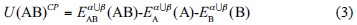In this manner, the three terms on the right hand side of Eq. (3) are calculated using the same basis set. The BSSE is known as the difference between the IPESs computed by Eqs. (2) and (3)U(AB)CP is a consistent method for correction of the IPES, U, with α, ∪ β. For B, A and B systems, α, ∪ β is a balanced basis set. The magnitude of these parameters are determined by the second virial coefficient, B2, that calculated using the IPES, U(r), (Equation (5)) ,where r is the distance separation of two components, T is temperature and NA is the Avogadro constant.

Results and Discussion

The ab initio and DFT methods are applied for exploring bond lengths, bond angles and dihedral angles (Table 1). A good agreement was found for the optimized geometrical parameters between B3LYP/6-31G(d) and B3LYP/aug-cc-pVDZ levels of theory.

For determining the IPES, U(r), of the cubic N8 dimer, the approaching of monomers from the far side based on the edge-face and face-face orientations were done. Two different orientations of N8 dimer are shown in Fig. 1. Thermodynamic properties of N8 and N8 dimer clusters in two different face-face and edge-face orientations at the cited computational levels are listed in Table 2. The obtained thermodynamic properties for edge-face orientation show good agreement between the both 6-31G(d) and aug-cc-pVDZ basis sets with B3LYP method, but with aug-cc-pVDZ basis set the edge-edge orientation was not obtained.

Fig. 2 and Fig. 3 show the calculated IPES, U(r), as a function of distance separation between the centers of N8-N8 system in the two different orientations using HF/3-21G and B3LYP/6-31G(d) levels, respectively. Fig. 4 shows the calculated IPES, U(r), as a function of distance separation between the centers of the N8-N8 in the edge-face orientation using B3LYP/aug-cc-pVDZ. In Figures 2, 3 and 4, the calculated IPES are compared with Lenard-Jones (12-6) potential with the BSSE corrected (CP) and uncorrected (NCP). The difference of Lenard-Jones (12-6) with computed data is very large. Therefore, this is a clear sign that Lenard-Jones (12-6) potential is "not" a proper function to fit the calculated data for N8-N8 supermolecule. The IPESs, U(r), strongly depend on the BSSE corrections. The IPESs corrected for the BSSE are energetically much lower than the potential energy calculated without the BSSE correction. In particular, the BSSE correction is important for calculating the IPESs with small basis sets.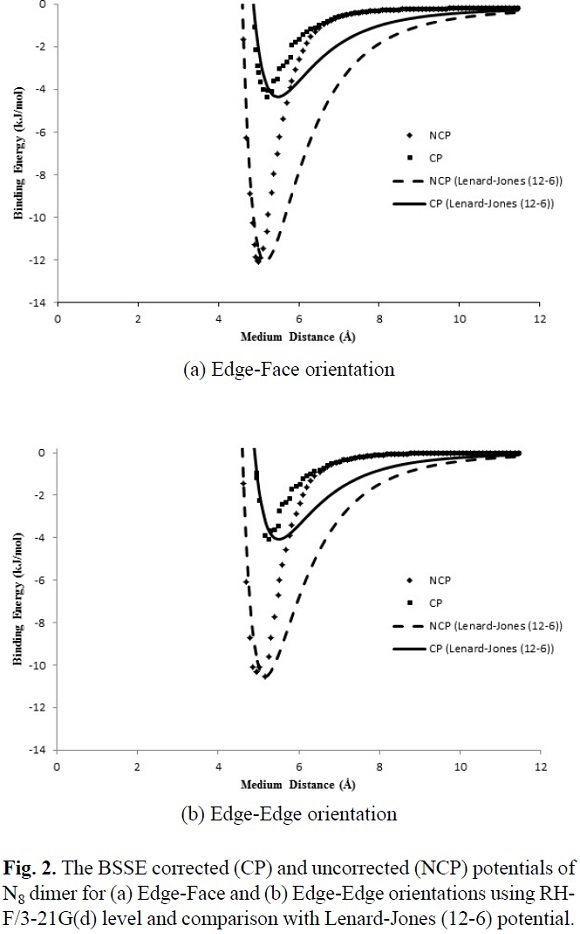It is seen from comparison of edge-face and face-face orientations in Figures 2 and 3 that the orientations and separation distances between the centers of N8-N8 clusters are fairly effective on the position of minimum, depth and width of the calculated potential well of the IPES curves, especially at HF/3-21G(d) level (Fig. 2). Figures 2 and 3 show that the edge-face orientation has the IPES curve with the largest value of De (De= - Eint(Re)= - Emin)2, at the HF/3-21G(d) level of theory; whereas, at the B3LYP/6-31G(d) level of theory, both of orientations have relatively similar potential energy curves. Also based on the position of the minimum point (Re) of the potential energy curves, the computed IPESs can be further compared. These quantities are very sensitive to the values of the separation distance of N8-N8 and different orientations of N8 monomers to each other that is applied in the computational methods. In Table 3, the values of Re and De are tabulated for the edge-face and edge-edge orientations. The potential well depths of the calculated IPESs, without the BSSE correction at RHF/3-21G(d) level are 2.5 to 3 times more than the BSSE corrected potential, while these ratios for B3LYP/6-31G(d) are 2 to 2.5 times more than the BSSE corrected potential and also for B3LYP/aug-cc-pVDZ level is less than 2 times more than the BSSE corrected potential.

In this work, the IPES, U(r), for the cubic N8 dimer system, was fitted to the Lennard-Jones (12-6) model (Equation (6)), which can be expressed as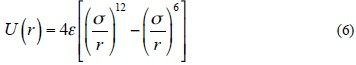where r is the separation distance between the centers of N8-N8, s is the size parameter and e is the depth of Lennard-Jones function. We fitted the adjustable parameters s and e for two different orientations with the ab initio HF/3-21G(d) and B3LYP/6-31G(d) interaction energies, whereas these parameters are obtained for edge-face orientation at B3LYP/aug-cc-pVDZ level. In Table 3, the values of the adjustable IPES parameters are tabulated for various orientations. Also, the fitted curves of the calculated IPESs to the Lennard-Jones (12-6) potential with the BSSE corrected (CP) and uncorrected (NCP), are shown in Figures 2, 3 and 4. The fitted IPESs to the Lennard-Jones (12-6) potential are not completely compatible with the curves calculated ISEPs at HF/3-21G(d), B3LYP/6-31G(d) and B3LYP/aug-cc-pVDZ levels. In addition, in this research, we demonstrated that it is possible to obtain a IPES, U(r), for the cubic N8 dimer by quantum mechanical calculations fitted to the Lennard-Jones (22-11) potential, (Equation (7)), which can be expressed aswhere the adjustable parameters e and s in Equation (7) are the same as the Lennard-Jones (12-6) model are tabulated in Table 3. The calculated IPES curves at HF/3-21G(d) and B3LYP/6-31G(d) levels are fitted to the Lennard-Jones (22-11) potential with the BSSE corrected (CP) and uncorrected (NCP) potential energy surfaces, as shown in Figures 5, 6 and 7. The IPESs fitted to the Lennard-Jones (22-11) potential are completely compatible with the curves of the calculated ISPs. Also, from the known U(r) formula, the second virial coefficients, B2, are estimated for the cubic N8 dimer system with the BSSE corrected (CP) and uncorrected (NCP) by quantum mechanical calculations. We calculated the second virial coefficients with the Lennard-Jones (12-6) and (22-11) potentials using Equation (5) and then tabulated the results for a range of temperatures from 298 to 1000 K in Table 4. However the equation (5) is valid if the systems is "spherical" (like an atom) but for this research we assumed N8 molecule is almost spherical. In Figures 8 and 9, the computed values of the second virial coefficients for selected temperatures (Table 4) are plotted.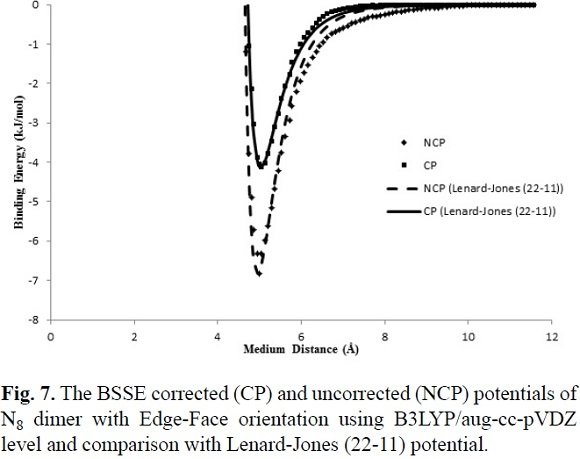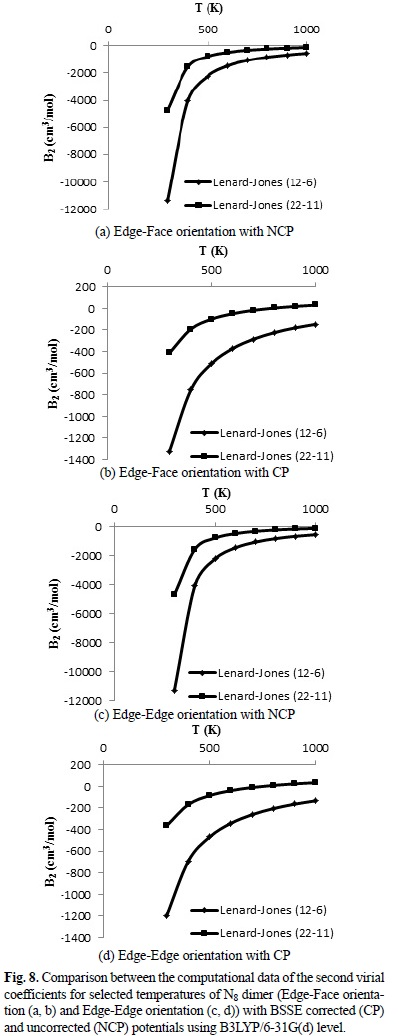We considered the BSSE corrections to yield the following systematic results. The obtained second virial coefficients (B2) with the Lenard-Jones (12-6) potential in the range of 298 to 1000 K with the BSSE corrected (CP) and uncorrected (NCP) potential energy surface are negatives, i.e. the attractive forces are dominated in the all range of 298 to 1000 K. Negative and positive second virial coefficients (B2) imply dominance of attractive and repulsive forces, respectively between N8 clusters. When we use the Lennard-Jones (22-11) potential, the obtained second virial coefficients in the range of 298 to 1000 K with the BSSE uncorrected (NCP) are negative, i.e. the attractive forces are dominated in the all range of 298 to 1000 K. While B2 computed with the BSSE corrected (CP) potential energy surface are varied from negative (approximately for a range of temperatures from 298 to 600 K) to positive (approximately for a range of temperatures from 700 to 1000 K) values.

Conclusion

In this research the calculated IPESs were systematically studied for cubic N8 dimer at the edge-face and edge-edge orientations applying both Hartree-Fock and DFT methods. The edge-face and edge-edge orientations were scanned at the HF/3-21G(d) and B3LYP/6-31G(d) levels but the edge-face is the only orientation that was obtained at B3LYP/aug-cc-pVDZ level. It was demonstrated that the BSSE corrections significantly affect the quality of calculated potentials. From this survey several significant conclusions about applying the current theoretical methods can be extracted to generate the IPESs.

Although in this study, we do not apply the long-range intermolecular interactions by DFT methods with dispersion-corrected and meta-hybrid functional and also MP2 methods with correlation energy for weakly bound systems (in van der Waals interactions), the results yield attractive potentials for the cubic N8 dimer system. The IPESs fitted to the Lennard-Jones (12-6) potential are not completely compatible with the curves of calculated IPESs at HF/3-21‏G(d), B3LYP/6-31G(d) and B3LYP/aug-cc-pVDZ levels, while the IPESs fitted to the Lennard-Jones (22-11) potential are completely compatible with the curves of the calculated IPESs.

Our calculated Lennard-Jones (22-11) potential with the BSSE corrected (CP) from 298 ~ 600 K show that the attractive forces are dominated and from 700 ~ 1000 K relatively repulsive forces are dominated.

These conclusions can be useful for subsequent studies on thermal decomposition and detonation of the cubic N8 as a high energy density material.

Acknowledgement

The authors are grateful to Dr. S. Shahbazian and Dr. C. Foroutan-Nejad for their helpful comments and valuable suggestions that helped the authors to improve the quality of this communication.

References

1. Hammerl, A.; Klapotke, T. M.; Schwerdtfeger, P. Chem. Eur. J. 2003, 9, 5511-5519.         [ Links ]

2. Christe, K. O. Prop. Explos. Pyrotech. 2007, 32, 194-204.         [ Links ]

3. Najafpour, J.; Foroutan-Nejad, C.; Shafiee, G. H.; Kordi-Peykani, M. Computational and Theoretical Chemistry. 2011, 974, 86-91.         [ Links ]

4. Dixon, D. A.; Feller, D.; Christe, K. O.; Wilson, W. W.; Vij, A.; Vij, V.; Jenkins, H. D. B.; Olson, R. M.; Gordon, M. S. J. Am. Chem. Soc. 2004, 126, 834-843.         [ Links ]

5. Fau, S.; Wilson, K. J.; Bartlett, R. J. J. Phys. Chem. A 2002, 106, 4639-4644.         [ Links ]

6. Ha, T. -K.; Suleimenov, O.; Nguyen, M. T. Chem. Phys. Lett. 1999, 315, 327-334.         [ Links ]

7. Nguyen, M. T. Coord. Chem. Rev. 2003, 244, 93-113.         [ Links ]

8. Cheng, L. P.; Li, S.; Li, Q. S. Int. J. Quant. Chem. 2004, 97, 933-943.         [ Links ]

9. Gu, J. -D.; Chen, K. -X.; Jiang, H. -L.; Chen, J. -Z.; Ji, R. -Y.; Ren, Y.; Tian, A. -M. J. Mol. Struct. (THEOCHEM). 1998, 428, 183-188.         [ Links ]

10. Gagliardi, L.; Evangelisti, S.; Roos, B. O.; Widmark, P. -O. J. Mol. Struct. (THEOCHEM) .1998, 428, 1-8.         [ Links ]

11. Zhou, H.; Zheng, W.; Wang, X.; Ren, Y.; Wong, N. -B.; Shu, Y.; Tian, A. J. Mol. Struct. (THEOCHEM). 2005, 732, 139-148.         [ Links ]

12. Sharma, H.; Garg, I.; Dharamvir, K.; Jindal, V. K. J. Phys. Chem. C. 2010, 114, 9153-9160.         [ Links ]

13. Chung, G.; Schmidt, M. W.; Gordon, M. S. J. Phys. Chem. A. 2000, 104, 5647-5650.         [ Links ]

14. Hirshberg, B.; Gerber, R. B.; Krylov, A. I. Nature Chemistry. 2014, 6, 52-56.         [ Links ]

15. Christe, K. O.; Wilson, W. W.; Sheehy, J. A.; Boatz, J. A. Angew. Chem. Int. Ed. 1999, 38, 2004-2009.         [ Links ]

16. Vij, A.; Wilson, W. W.; Vij, V.; Tham. F. S.; Sheehy, J. A.; Christe, K. O. J. Am. Chem. Soc. 2001, 123, 6308-6313.         [ Links ]

17. Vij, A.; Pavlovich, J. G.; Wilson, W. W.; Vij, V.; Christe, K. O. Angew. Chem. Int. Ed. 2002, 41, 3051-3054.         [ Links ]

18. Ostmark, H.; Wallin, S.; Brinck, T.; Carlqvist, P.; Claridge, R.; Hedlund, E.; Yudina, L. Chem. Phys. Lett. 2003, 379, 539-546.         [ Links ]

19. Wilson, W. W.; Vij, A.; Vij, V.; Bernhardt, E.; Christe, K. O. Chem. Eur. J. 2003, 9, 2840-2844.         [ Links ]

20. Butler, R. N.; Stephens, J. C.; Burke, L. A. Chem. Commun. 2003, 8, 1016-1017.         [ Links ]

21. Schroer, T.; Haiges, R.; Schneider, S.; Christe, K. O. Chem. Commun. 2005, 12, 1607-1609.         [ Links ]

22. Butler, R. N.; Hanniffy, J. M.; Stephens, J. C.; Burke, L. J. Org. Chem. 2008, 73, 1354-1364.         [ Links ]

23. Engelke, R.; Stine, J. R. J. Phys. Chem. 1990, 94, 5689-5694.         [ Links ]

24. Lauderdale, W. J.; Stanton, J. F.; Bartlett, R. J. J. Phys. Chem. 1992, 96, 1173-1178.         [ Links ]

25. Leininger, M. L.; Sherrill C. D.; Schaefer, III, H. J. Phys. Chem. 1995, 99, 2324-2328.         [ Links ]

26. Gagliardi, L.; Evangelistil, S.; Widmark, P. O.; Roos, B. O. Theor. Chem. Acc. 1997, 97, 136-142.         [ Links ]

27. Smith, L. R. J. Chem. Ed. 1978, 55, 569-570.         [ Links ]

28. March, J. Advanced Organic Chemistry New York, Wiley, 1985.         [ Links ]

29. Eaton, P. E.; Cole, T. W. J. Am. Chem. Soc. 1964, 86, 3157-3158.         [ Links ]

30. Li, A. H. –T.; Chaoa, S. D. J. Chem. Phys. 2006, 125, 094312.         [ Links ]

31. Boys, S. F.; Bernardi, F. Mol. Phys. 1970, 19, 553-566.         [ Links ]

32. Monajjemi, M.; Khaleghian, M.; Mollaamin, F. Molecular Simulation. 2010, 36, 865-870.         [ Links ]

33. Shi, Y.; Brenner, D. W. J. Chem. Phys. 2007, 127, 134503.         [ Links ]

34. Becke, A. D. J. Chem. Phys. 1993, 98, 5648-5652.         [ Links ]

35. Becke, A. D. Phys. Rev. A. 1998, 38, 3098-3100.         [ Links ]

36. Lee, C.; Yang, W.; Parr, R. G. Phys. Rev. B. 1988, 37, 785-789.         [ Links ]

37. Woon, D. E.; Dunning Jr, T. H. J. Chem. Phys. 1993, 98, 1358-1371.         [ Links ]

38. Moller, C.; Plesset, M. S. Phys. Rev. 1934, 46, 618-622.         [ Links ]

39. Saebo, S.; Almlof, J. Chem. Phys. Lett. 1989, 154, 83-89.         [ Links ]

40. Hehre, W. J.; Ditchfield, R.; Pople, J. A. J. Chem. Phys. 1972, 56, 2257-2261.         [ Links ]

41. Clark, T.; Chandrasekhar, J.; Spitznagel, G. W.; Schleyer, P. V. R. J. Comp. Chem. 1983, 4, 294-301.         [ Links ]

42. Hariharan, P. C. Pople, J. A. Theor. Chim. Acta. 1973, 28, 213-222.         [ Links ]

43. Spartan '10, Version 1.1.0, Deppmeier, B. J.; Driessen, A. J.; Hehre, T. S.; Hehre, W. J.; Johnson, J. A.; Klunzinger, P. E.; Leonard, J. M.; Pham, I. N., Pietro, W. J.; Yu, Jianguo, Irvine, CA, Wavefunction, Inc., 2011.         [ Links ]

44. Sordo, J. A. J. Mol. Struct. (THEOCHEM) 2001, 537, 245-251.         [ Links ]

45. Mierzecki, R. Intermolecular Interactions Warsaw, PWN, 1974.         [ Links ]Todo el contenido de esta revista, excepto dónde está identificado, está bajo una Licencia Creative Commons